11 de Dec de 2019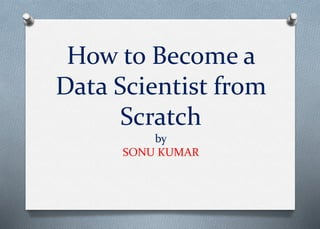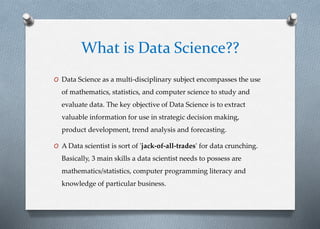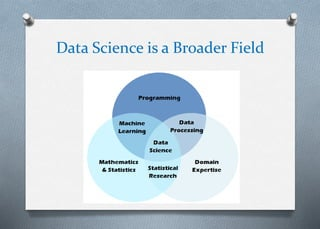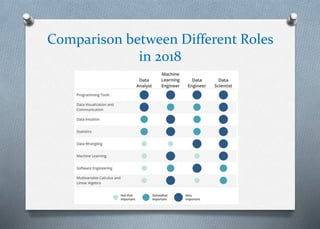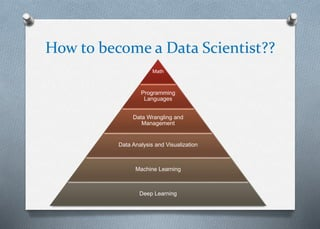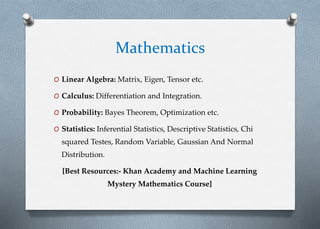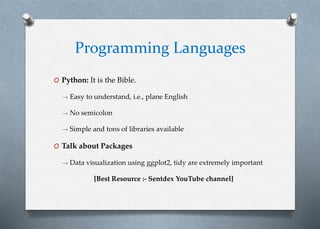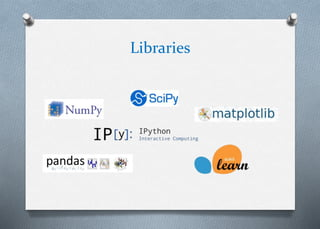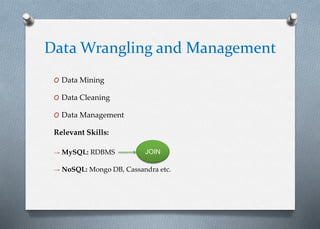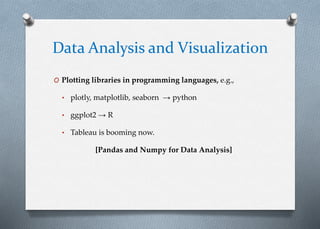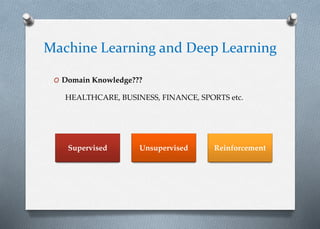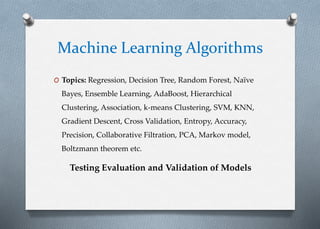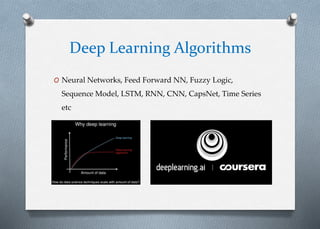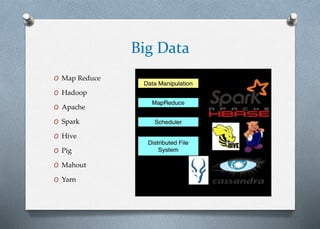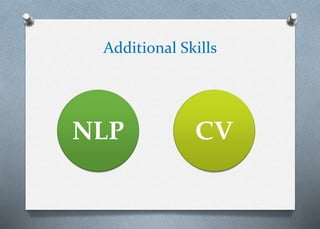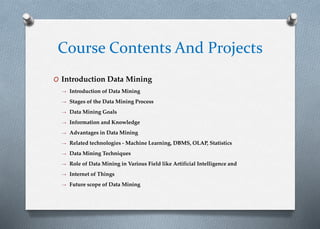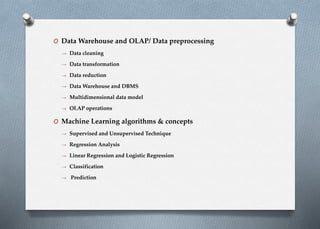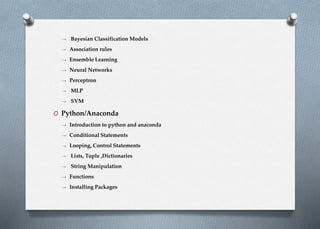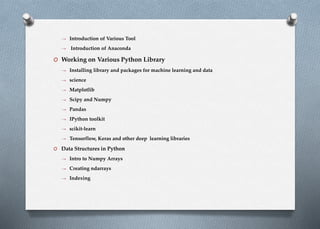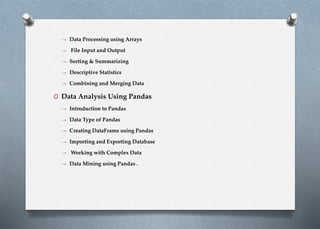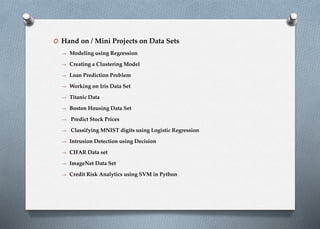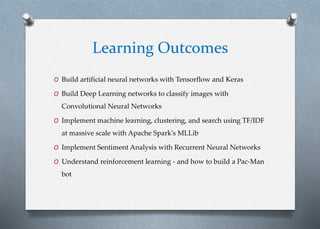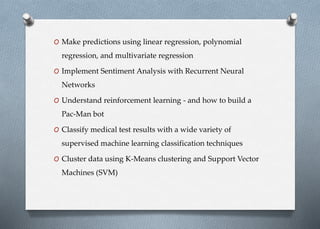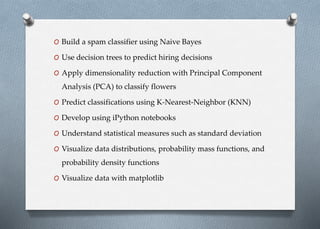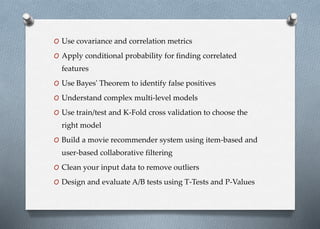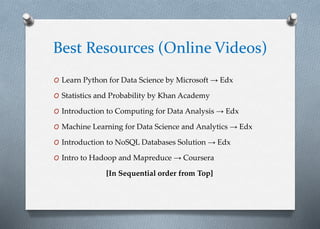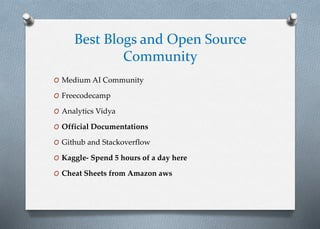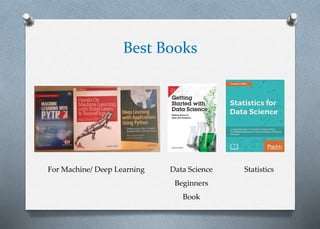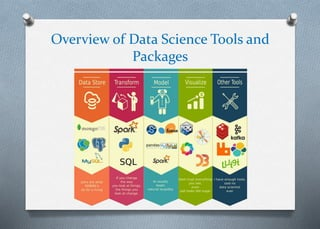1 de 30

• 1. How to Become a Data Scientist from Scratch by SONU KUMAR
• 2. What is Data Science?? O Data Science as a multi-disciplinary subject encompasses the use of mathematics, statistics, and computer science to study and evaluate data. The key objective of Data Science is to extract valuable information for use in strategic decision making, product development, trend analysis and forecasting. O A Data scientist is sort of 'jack-of-all-trades' for data crunching. Basically, 3 main skills a data scientist needs to possess are mathematics/statistics, computer programming literacy and knowledge of particular business.
• 3. Data Science is a Broader Field
• 4. Comparison between Different Roles in 2018
• 5. How to become a Data Scientist?? Math Programming Languages Data Wrangling and Management Data Analysis and Visualization Machine Learning Deep Learning
• 6. Mathematics O Linear Algebra: Matrix, Eigen, Tensor etc. O Calculus: Differentiation and Integration. O Probability: Bayes Theorem, Optimization etc. O Statistics: Inferential Statistics, Descriptive Statistics, Chi squared Testes, Random Variable, Gaussian And Normal Distribution. [Best Resources:- Khan Academy and Machine Learning Mystery Mathematics Course]
• 7. Programming Languages O Python: It is the Bible. → Easy to understand, i.e., plane English → No semicolon → Simple and tons of libraries available O Talk about Packages → Data visualization using ggplot2, tidy are extremely important [Best Resource :- Sentdex YouTube channel]
• 8. Libraries
• 9. Data Wrangling and Management O Data Mining O Data Cleaning O Data Management Relevant Skills: → MySQL: RDBMS → NoSQL: Mongo DB, Cassandra etc. JOIN
• 10. Data Analysis and Visualization O Plotting libraries in programming languages, e.g., • plotly, matplotlib, seaborn → python • ggplot2 → R • Tableau is booming now. [Pandas and Numpy for Data Analysis]
• 11. Machine Learning and Deep Learning O Domain Knowledge??? HEALTHCARE, BUSINESS, FINANCE, SPORTS etc. Supervised Unsupervised Reinforcement
• 12. Machine Learning Algorithms O Topics: Regression, Decision Tree, Random Forest, Naïve Bayes, Ensemble Learning, AdaBoost, Hierarchical Clustering, Association, k-means Clustering, SVM, KNN, Gradient Descent, Cross Validation, Entropy, Accuracy, Precision, Collaborative Filtration, PCA, Markov model, Boltzmann theorem etc. Testing Evaluation and Validation of Models
• 13. Deep Learning Algorithms O Neural Networks, Feed Forward NN, Fuzzy Logic, Sequence Model, LSTM, RNN, CNN, CapsNet, Time Series etc
• 14. Big Data O Map Reduce O Hadoop O Apache O Spark O Hive O Pig O Mahout O Yarn
• 15. Additional Skills NLP CV
• 16. Course Contents And Projects O Introduction Data Mining → Introduction of Data Mining → Stages of the Data Mining Process → Data Mining Goals → Information and Knowledge → Advantages in Data Mining → Related technologies - Machine Learning, DBMS, OLAP, Statistics → Data Mining Techniques → Role of Data Mining in Various Field like Artificial Intelligence and → Internet of Things → Future scope of Data Mining
• 17. O Data Warehouse and OLAP/ Data preprocessing → Data cleaning → Data transformation → Data reduction → Data Warehouse and DBMS → Multidimensional data model → OLAP operations O Machine Learning algorithms & concepts → Supervised and Unsupervised Technique → Regression Analysis → Linear Regression and Logistic Regression → Classification → Prediction
• 18. → Bayesian Classification Models → Association rules → Ensemble Learning → Neural Networks → Perceptron → MLP → SVM O Python/Anaconda → Introduction to python and anaconda → Conditional Statements → Looping, Control Statements → Lists, Tuple ,Dictionaries → String Manipulation → Functions → Installing Packages
• 19. → Introduction of Various Tool → Introduction of Anaconda O Working on Various Python Library → Installing library and packages for machine learning and data → science → Matplotlib → Scipy and Numpy → Pandas → IPython toolkit → scikit-learn → Tensorflow, Keras and other deep learning libraries O Data Structures in Python → Intro to Numpy Arrays → Creating ndarrays → Indexing
• 20. → Data Processing using Arrays → File Input and Output → Sorting & Summarizing → Descriptive Statistics → Combining and Merging Data O Data Analysis Using Pandas → Introduction to Pandas → Data Type of Pandas → Creating DataFrame using Pandas → Importing and Exporting Database → Working with Complex Data → Data Mining using Pandas .
• 21. O Hand on / Mini Projects on Data Sets → Modeling using Regression → Creating a Clustering Model → Loan Prediction Problem → Working on Iris Data Set → Titanic Data → Boston Housing Data Set → Predict Stock Prices → Classifying MNIST digits using Logistic Regression → Intrusion Detection using Decision → CIFAR Data set → ImageNet Data Set → Credit Risk Analytics using SVM in Python
• 22. Learning Outcomes O Build artificial neural networks with Tensorflow and Keras O Build Deep Learning networks to classify images with Convolutional Neural Networks O Implement machine learning, clustering, and search using TF/IDF at massive scale with Apache Spark's MLLib O Implement Sentiment Analysis with Recurrent Neural Networks O Understand reinforcement learning - and how to build a Pac-Man bot
• 23. O Make predictions using linear regression, polynomial regression, and multivariate regression O Implement Sentiment Analysis with Recurrent Neural Networks O Understand reinforcement learning - and how to build a Pac-Man bot O Classify medical test results with a wide variety of supervised machine learning classification techniques O Cluster data using K-Means clustering and Support Vector Machines (SVM)
• 24. O Build a spam classifier using Naive Bayes O Use decision trees to predict hiring decisions O Apply dimensionality reduction with Principal Component Analysis (PCA) to classify flowers O Predict classifications using K-Nearest-Neighbor (KNN) O Develop using iPython notebooks O Understand statistical measures such as standard deviation O Visualize data distributions, probability mass functions, and probability density functions O Visualize data with matplotlib
• 25. O Use covariance and correlation metrics O Apply conditional probability for finding correlated features O Use Bayes' Theorem to identify false positives O Understand complex multi-level models O Use train/test and K-Fold cross validation to choose the right model O Build a movie recommender system using item-based and user-based collaborative filtering O Clean your input data to remove outliers O Design and evaluate A/B tests using T-Tests and P-Values
• 26. Best Resources (Online Videos) O Learn Python for Data Science by Microsoft → Edx O Statistics and Probability by Khan Academy O Introduction to Computing for Data Analysis → Edx O Machine Learning for Data Science and Analytics → Edx O Introduction to NoSQL Databases Solution → Edx O Intro to Hadoop and Mapreduce → Coursera [In Sequential order from Top]
• 27. Best Blogs and Open Source Community O Medium AI Community O Freecodecamp O Analytics Vidya O Official Documentations O Github and Stackoverflow O Kaggle- Spend 5 hours of a day here O Cheat Sheets from Amazon aws
• 28. Best Books For Machine/ Deep Learning Data Science Beginners Book Statistics
• 29. Overview of Data Science Tools and Packages
• 30. Thank You